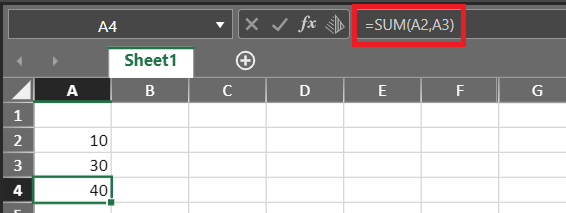# Formula Bar

The Formula Bar is positioned above the Tabulate worksheet.In the above example, a formula (=SUM(A2,A3)) has been inserted in cell A4. When this cell is selected, the formula is displayed in the Formula Bar.

You can subsequently edit any formula displayed in this bar.